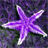# simple table calculation in modelbuilder

3875
3
04-28-2015 02:37 PMOccasional Contributor II

Any suggestions of a tool that permits you to divide one table by another table in model builder.  We can use field calculator to perform calculations of values within one table but not if in different tables?

Tags (4)
3 Repliesby
Occasional Contributor II

Perhaps joining the tables first to create a new table should first be done. Then field calculator is possible again.by
Frequent Contributor

Hi Warren, simply join the two tables together using the Join Field tool (ArcGIS Help (10.2, 10.2.1, and 10.2.2)

Use a common Id field as the join_field, and you can join whichever of your fields from the second table you need to use in calculations.byMVP Esteemed Contributor

I'm assuming the model builder element labeled "this value is a constant" means that it is a one-row table, with a value you are dividing rows by in the other table.

If that's the case, you could use the Get Field Value tool to pull the value out from your one-row table into a model variable. Then you can use Calculate Field, including that model element in the expression like this:

`!VFIELD! / %field value%`

where "field value" is the model element output from the Get Field Value tool.

By the way, I always use the PYTHON parser (instead of the default VB parser) because it is the only one that works in both foreground and background processing.